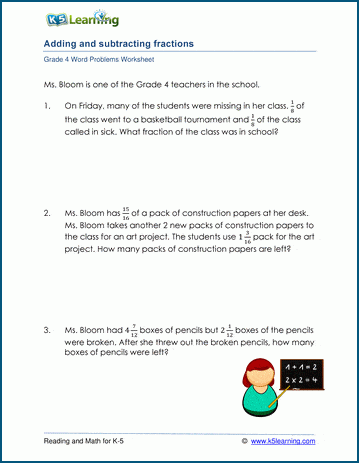# Adding & subtracting fractions word problems

## Word problem worksheets: Addition & subtraction of fractions

Below are three versions of our grade 4 math worksheet on adding and subtracting fractions and mixed numbers.  All fractions have like denominators.  Some problems will include irrelevant data so that students have to read and understand the questions, rather than simply recognizing a pattern to the solutions.  These worksheets are pdf files.## More word problem worksheets

Explore all of our math word problem worksheets, from kindergarten through grade 5.

## What is K5?

K5 Learning offers reading and math worksheets, workbooks and an online reading and math program for kids in kindergarten to grade 5.  We help your children build good study habits and excel in school.